Engineer Talk

# Why use a Diode with a Heater?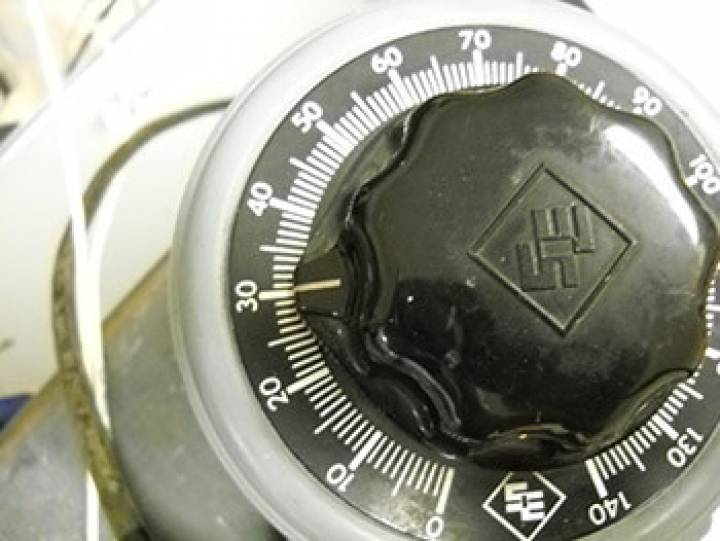## Here’s why:

Sometimes, when all the design work is done, the heating element is too fragile for either the assembly process, or field operation. This can occur when making a low wattage heater that uses a high voltage. In these cases the element needs to be more robust but the design won’t allow it. One solution is to use a diode in series with the heating element. The diode will reduce the applied energy to the heater for any given voltage and allow a larger lower resistance heating element to be used. Naturally this is not applicable to DC powered heaters.

## How it works:

A diode blocks half of each cycle in the incoming AC voltage wave. This means that there is no power dissipated or applied during those half cycles. So while the applied voltage remains the same as the application requirements, the power reaching the heating element is cut in half. As a result a larger tougher element with half the resistance is now useable to meet the application wattage.

## Engineering Details:

Vrms is the application supply voltage - (Vpk = Vrms * 1.414)

Vrms’ is the volts RMS applied with a diode

W is the application wattage

W’ is the wattage with a diode

R is the original calculated resistance

R’ is the required resistance to get the same wattage with a diode

Neglecting the .7v diode junction drop for simplicity, we get the following change in voltage applied to the heating element:

Vrms’ = Vrms *.707 or equivalently Vrms’ = Vpk / 2

Given that W= V^2 / R the result is W’ = ½ W

So, R’ will have to be half of the original design R to get the same wattage with a diode.

### Design notes:

The diode must be able to withstand the peak reverse voltage for the life of the heater. Any diode selected should probably have a Reverse Breakdown Voltage (Vbr) of at least twice the(not the RMS ) of the application voltage. The diode must be able to withstand the peak heater current, for the diode that’s the rated Forward Current or If. Therefore If must be greater than Ipk, where Ipk = Vpk / R’.

## Here’s the Catch:

First, once the heater is assembled you can’t check the coil resistance through the diode. If you need to check it you must find a way to test that doesn’t include the diode in the circuit. Second, the calculations shown above seem simple enough but you cannot confirm them with a hand held meter. After contacting a manufacturer of True RMS meters, they verified that no hand held meter will produce accurate readings with half the waveform missing since they cannot do the actual integration of the waveform. So you have to trust the calculations or rely on an oscilloscope to measure the results.

## Example:

The photos below illustrate the use of an oscilloscope and the problems with a handheld meter:

Variac setting showing 30 Vac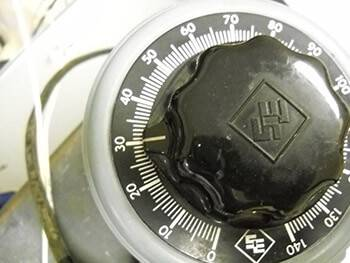Rectified waveform:

Peak voltage shown in upper right (30Vac * 1.414 = 42.4 vac).The scope can integrate the waveform to produce an accurate RMS result:

RMS voltage shown in upper right

((42.4 - 0.7) / 2) = 20.85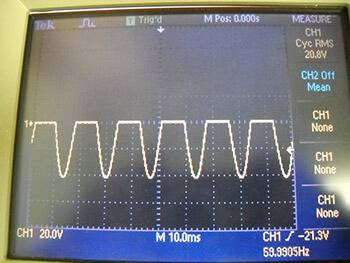True RMS meter reading Vac and Vdc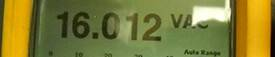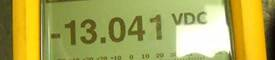## In Conclusion:

While there are certainly some testing difficulties to overcome when using a diode, it is an easy way to make a fragile heating element more robust.

Tutco-Farnam Custom Products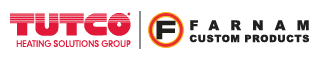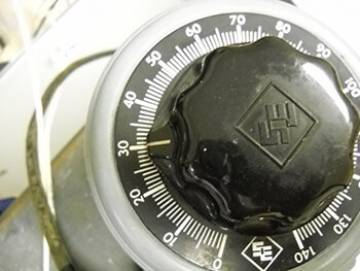Calculators
Calculators +